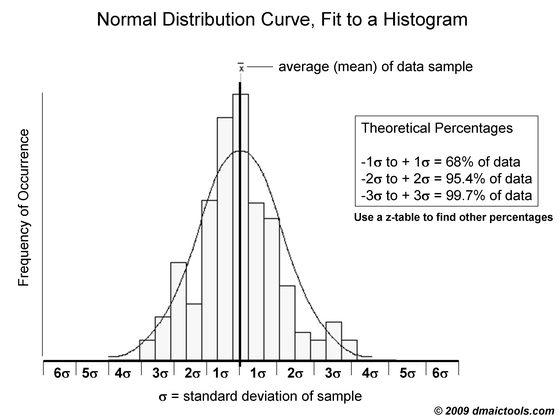# Normal Probability Curve

A normal probability curve shows the theoretical shape of a normally distributed histogram.  The shape of the normal probability curve is based on two parameters:  mean (average) and standard deviation (sigma).  The equation behind the normal probability curve itself is fairly complex, but defect-rate predictions for Six Sigma projects are made easily by using normal probability tables (commonly known as z-tables – see the PPM calculator and z-table Excel files on our excel templates page).  The example below shows a a normal probability curve, fit to a histogram using statistical software.

In the DMAIC world, normal distributions are used for making predictions and conducting certain hypothesis tests.  The 3.4 DPM rate associated with Six Sigma processes is based on the normal distribution curve.